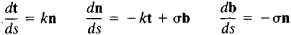# Frénet Formulas

The following article is from The Great Soviet Encyclopedia (1979). It might be outdated or ideologically biased.

## Frénet Formulas

formulas giving the derivatives, with respect to arc length S, of the unit tangent vector t, the unit normal vector n, and the unit binormal vector b of the arbitrary curve L along the vectors t, n, and b. Ifk and σ are the curvature and torsion of L, then the Frénet formulas have the formFrénet formulas are used to study the differential-geometric properties of curves and, in kinematics, to investigate the motion of a mass point along a curvilinear trajectory.

The French mathematician F. Frénet used the formulas as early as 1847 but did not publish them until 1852. They were first published by the French mathematician J. Serret in 1851 and consequently are often called the Serret-Frénet, or Frénet-Serret, formulas.